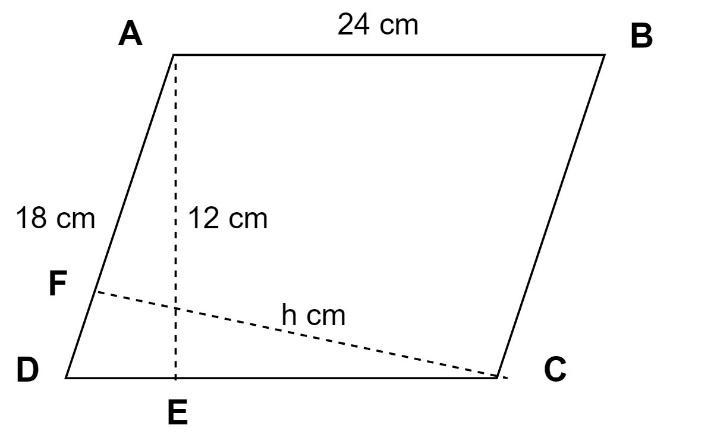Courses
Courses for Kids
Free study material
Free LIVE classes
More# Two adjacent sides of a parallelogram are 24cm and 18cm. If the distance between the longer sides is 12cm, find the distance between the shorter sides.A.9 cmB.16 cmC.24 cmD.Data insufficientVerified
327.9k+ views
Hint: First we need to draw the parallelogram to assign the given sides. Using the area of parallelogram, we can find the distance between the shorter sides.Given the parallelogram ABCD with AB=24cm and AD=18cm.
Projecting a perpendicular line from vertex A to base DC at point E.
Since the distance between two lines is the perpendicular distance between them.
And the distance between the longer sides of the parallelogram is given 12cm in the problem.
Hence, AE=12cm
We know that area of the parallelogram is given by
Area = Base x Height
For parallelogram ABCD, Base=AB=CD=24cm, as the opposite sides of a parallelogram are equal. Similarly, Height=AE=12cm.
Therefore, area of parallelogram ABCD $= {\text{AB}} \times {\text{AE = 24}} \times 12 = 288{\text{cm}}$.
We can also consider the area of the parallelogram ABCD by considering the shorter aside as the base.
Considering the base of the parallelogram ABCD as AD=BC=18cm.
Projecting a perpendicular line from vertex C to base AD at point F, we get Height CF=h cm.
Now again,
Area = Base x Height
$\Rightarrow 288 = {\text{AD}} \times {\text{CF}} \\ \Rightarrow 288 = 18 \times {\text{h}} \\ \Rightarrow {\text{h = }}\dfrac{{288}}{{18}} = 16{\text{cm}} \\$
Hence CF=h=16 cm.
Since the distance between two lines is the perpendicular distance between them.
Distance between the shorter sides of the parallelogram ABCD = CF= 16cm.
Hence option (B) is the correct answer.
Note: It is important to draw the figure in the problems like above in order to solve the problem easily and accurately. Properties of the parallelogram should be kept in mind in solving the problems like above.
Last updated date: 03rd Jun 2023
Total views: 327.9k
Views today: 6.84k